# Angle + reason - math problems

#### Number of problems found: 38

• Wheel gearA drive wheel of radius 2 is connected to a drive wheel of radius 1 by a pulley of length 17. What is the distance between the wheel axles?
• Ground levelThe temperature at ground level in Helsinki is 20°C lower than in London. The temperature in a basement flat in Helsinki is 5°C below the temperature at ground level. If it is -29°c in the basement, what is the temperature in London?
• AnnulusTwo concentric circles with radii 1 and 9 surround the annular circle. This ring is inscribed with n circles that do not overlap. Determine the highest possible value of n.
• Magnitude of angleWhat magnitude has an obtuse angle enclosed by the hands of clocks at 12:20 hours?
• A drone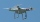A flying drone aimed the area for an architect. He took off perpendicularly from point C to point D. He was at the height of 300 m above ABC's plane. The drone from point D pointed at a BDC angle of 43°. Calculate the distance between points C and B in me
• Irregular pentagon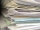A rectangle-shaped, 16 x 4 cm strip of paper is folded lengthwise so that the lower right corner is applied to the upper left corner. What area does the pentagon have?
• Circular railwayThe railway connects in a circular arc the points A, B, and C, whose distances are | AB | = 30 km, AC = 95 km, BC | = 70 km. How long will the track from A to C?
• Depth anglesAt the top of the mountain stands a castle, which has a tower 30 meters high. We see the crossroad in the valley from the top of the tower and heel at depth angles of 32° 50 'and 30° 10'. How high is the top of the mountain above the crossroad
• Each with each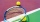Five pupils from 3A class played table tennis. How many matches did they play with each other?
• Special watchFero bought a special watch on the market. They have only one (minute) hand and a display that shows which angle between the hour and minute hand. How many hours was his watch shown - the minute hand points to number 2; the display shows 125°?
• Right triangle eq2Find the lengths of the sides and the angles in the right triangle. Given area S = 210 and perimeter o = 70.
• Rhombus 29One of the diagonals of a rhombus is equal to a side of the rhombus. Find the angles of the rhombus.
• ABCD squareIn the ABCD square, the X point lies on the diagonal AC. The length of the XC is three times the length of the AX segment. Point S is the center of the AB side. The length of the AB side is 1 cm. What is the length of the XS segment?
• CloudsApproximately at what height is the cloud we see under an angle of 26°10' and see the Sun at an angle of 29°15' and the shade of the cloud is 92 meters away from us?
• Four sides of trapezoidIn the trapezoid ABCD is |AB| = 73.6 mm; |BC| = 57 mm; |CD| = 60 mm; |AD| = 58.6 mm. Calculate the size of its interior angles.
• Time clockWhat are the angle of the hour hand and the minute hand if it is 0:40?
• Candy - MOGretel deploys to the vertex of a regular octagon different numbers from one to eight candy. Peter can then choose which three piles of candy give Gretel others retain. The only requirement is that the three piles lie at the vertices of an isosceles trian
• Triangle ABCTriangle ABC has side lengths m-1, m-2, m-3. What has to be m to be triangle a) rectangular b) acute-angled?
• Clock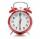What distance will pass end of 8 cm long hour hand for 15 minutes?
• Clocks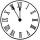What distance will describe the tip of minute hand 6 cm long for 20 minutes when we know the starting position with finally enclose hands each other 120°?

Do you have an exciting math question or word problem that you can't solve? Ask a question or post a math problem, and we can try to solve it.

We will send a solution to your e-mail address. Solved examples are also published here. Please enter the e-mail correctly and check whether you don't have a full mailbox.

Angle Problems. Reason - math problems.